NCERT Solutions for Class 9 Maths Chapter 13 Surface Areas and Volumes Ex 13.8

# NCERT Solutions for Class 9 Maths Chapter 13 Surface Areas and Volumes Ex 13.8

## NCERT Solutions for Class 9 Maths Chapter 13 Surface Areas and Volumes Ex 13.8

NCERT Solutions for Class 9 Maths Chapter 13 Surface Areas and Volumes Ex 13.8 are the part of NCERT Solutions for Class 9 Maths. Here you can find the NCERT Solutions for Chapter 13 Surface Areas and Volumes Ex 13.8.

## NCERT Solutions for Class 9 Maths Chapter 13 Surface Areas and Volumes Ex 13.8

Ex 13.8 Class 9 Maths Question 1.
Find the volume of a sphere whose radius is:
(i) 7 cm
(ii) 0.63 cm

(ii) Here, radius (r) = 0.63 m

Thus, the required volume is 1.05 m3 (approx.)

Ex 13.8 Class 9 Maths Question 2.
Find the amount of water displaced by a solid spherical ball of diameter:
(i) 28 cm
(ii) 0.21 m

Solution:
(i) Diameter of the ball = 28 cm
Radius of the ball (r) = 28/2 cm = 14 cm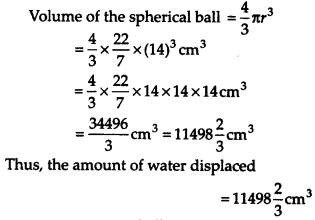(ii) Diameter of the ball = 0.21 m
Radius (r) = 0.21/2 m = 21/200 m

Ex 13.8 Class 9 Maths Question 3.
The diameter of a metallic ball is 4.2 cm. What is the mass of the ball, if the density of the metal is 8.9 g per cm3?

Solution:
Diameter of the metallic ball = 4.2 cm
Radius of the metallic ball (r) = 4.2/2 cm = 2.1 cm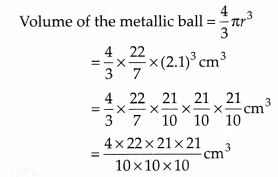Density of the metal = 8.9 g per cm3

Mass of the ball = 8.9 × [Volume of the ball]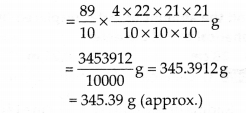Thus, the mass of ball is 345.39 g (approx.)

Ex 13.8 Class 9 Maths Question 4.
The diameter of the Moon is approximately one-fourth of the diameter of the Earth. What fraction of the volume of the Earth is the volume of the Moon?

Solution:
Let the diameter of the earth be 2r.
Radius of the earth = 2r/2 = r
Since, diameter of the moon = ¼ (Diameter of the earth)
Radius of the moon = ¼ (r) = r/4
Volume of the earth = 4/3 πr3 and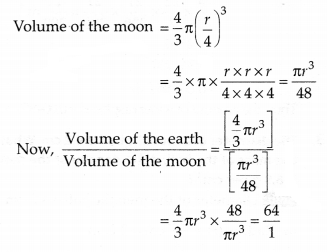Ex 13.8 Class 9 Maths Question 5.

How many litres of milk can a hemispherical bowl of diameter 10.5 cm hold?

Solution:
Diameter of the hemispherical bowl = 10.5 cm
Radius of the hemispherical bowl (r) = 10.5/2 cm = 105/20 cm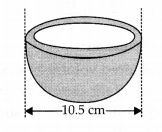Thus, the capacity of the bowl = 0.303 litres (approx.)

Ex 13.8 Class 9 Maths Question 6.
A hemispherical tank is made up of an iron sheet 1 cm thick. If the inner radius is 1 m, then find the volume of the iron used to make the tank.

Solution:
Inner radius (r) = 1 m
Thickness = 1 cm = 1/100 m = 0 .01 m

Outer radius (R) = 1 m + 0.01 m = 1.01 m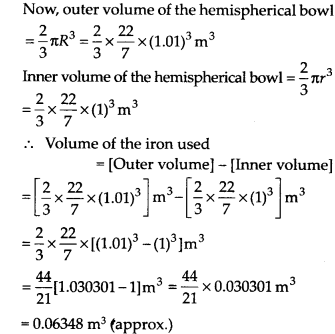Thus, the required volume of the iron used = 0.06348 m3 (approx.)

Ex 13.8 Class 9 Maths Question 7.
Find the volume of a sphere whose surface area is 154 cm2.

Solution:
Let ‘r’ be the radius of the sphere.
Surface area of the sphere = 4πr2
4πr2 = 154          [Given]

Ex 13.8 Class 9 Maths Question 8.
A dome of a building is in the form of a hemisphere. From inside, it was white washed at the cost of ₹498.96. If the cost of white washing is ₹2.00 per square metre, find the
(i) inside surface area of the dome,
(ii) volume of the air inside the dome.

Solution:
(i) Total cost of white-washing = ₹ 498.96
Cost of 1 m² of white-washing = ₹ 2
Area = Total/Cost/Cost of 1 m2 area = 498.96/2 = 249.48 m2
Thus, the required surface area of the dome is 249.48 m2.

(ii) Let ‘r’ be the radius of the hemispherical dome.
Inside surface area of the dome = 2πr2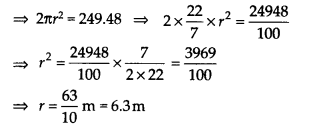Now, volume of air inside the dome = Volume of a hemisphere

Thus, the required volume of air inside the dome is 523.9 m3 (approx.).

Ex 13.8 Class 9 Maths Question 9.
Twenty-seven solid iron spheres, each of radius r and surface area S are melted to form a sphere with surface area S’. Find the
(i) radius r’ of the new sphere,
(ii) ratio of S and S’.

Solution:
(i) The radius of the small sphere is r.
Its volume = 4/3 πr3
Volume of 27 small spheres 27 × [4/3 πr3]
Let the radius of the new sphere be r’.
Volume of the new sphere = 4/3 π(r’)3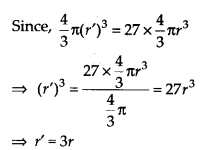Hence, the radius of the new sphere is 3r.

(ii) Surface area of a sphere = 4πr2
S = 4πr2 and S’ = 4π (3r)2             [
r’ = 3r]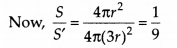Thus, S : S’ = 1 : 9

Ex 13.8 Class 9 Maths Question 10.
A capsule of medicine is in the shape of a sphere of diameter 3.5 mm. How much medicine (in mm3) is needed to fill this capsule?

Solution:
Diameter of the spherical capsule = 3.5 mm
Radius of the spherical capsule (r) = 3.5/2 mm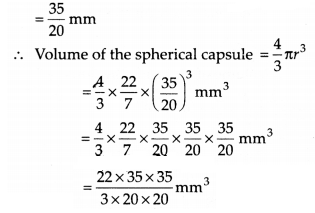= 22.45833 mm3

= 22.46 mm3 (approx.)

Thus, the required quantity of medicine = 22.46 mm3 (approx.)

You can also like these:

NCERT Solutions for Maths Class 10

NCERT Solutions for Maths Class 12